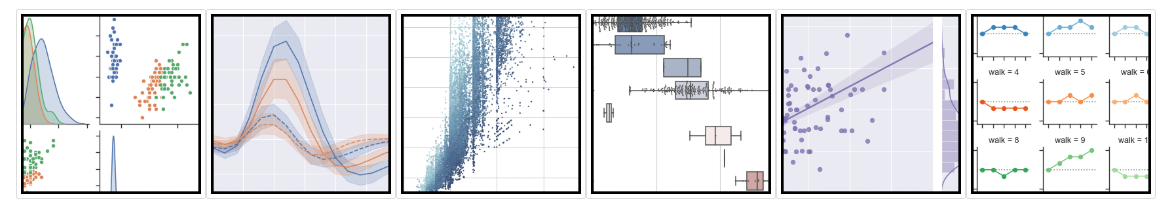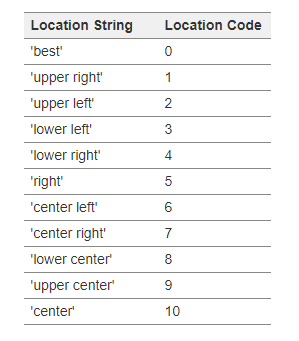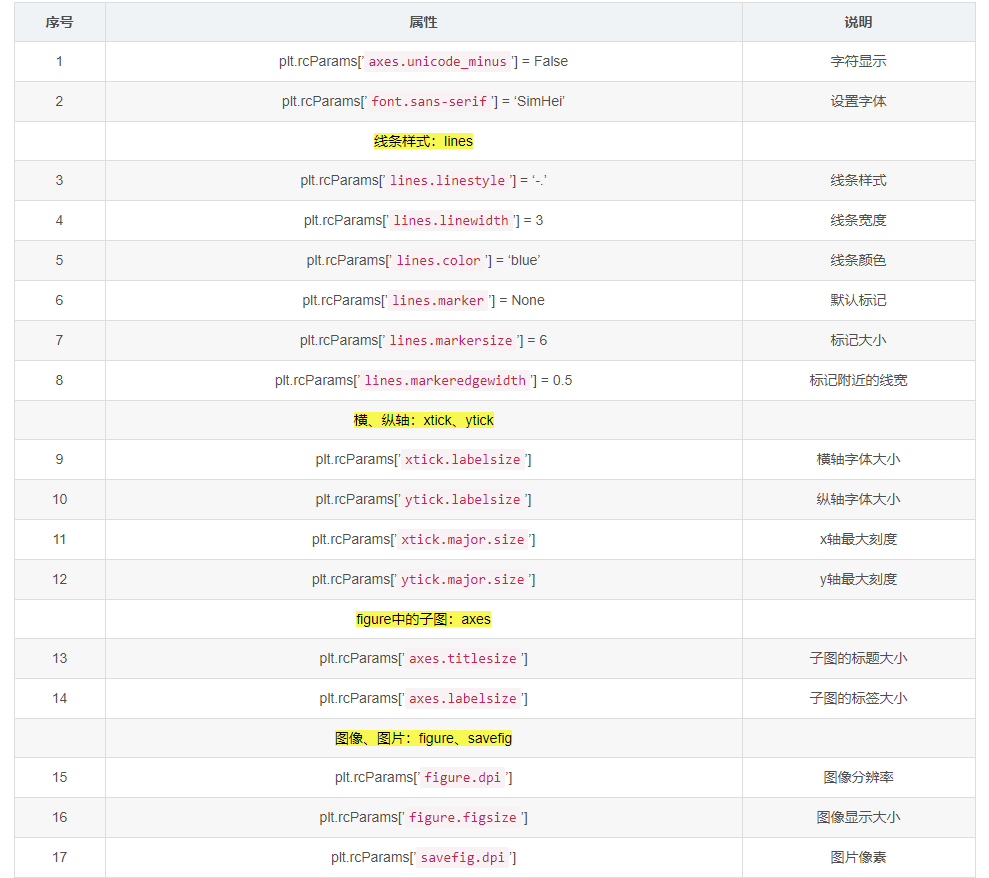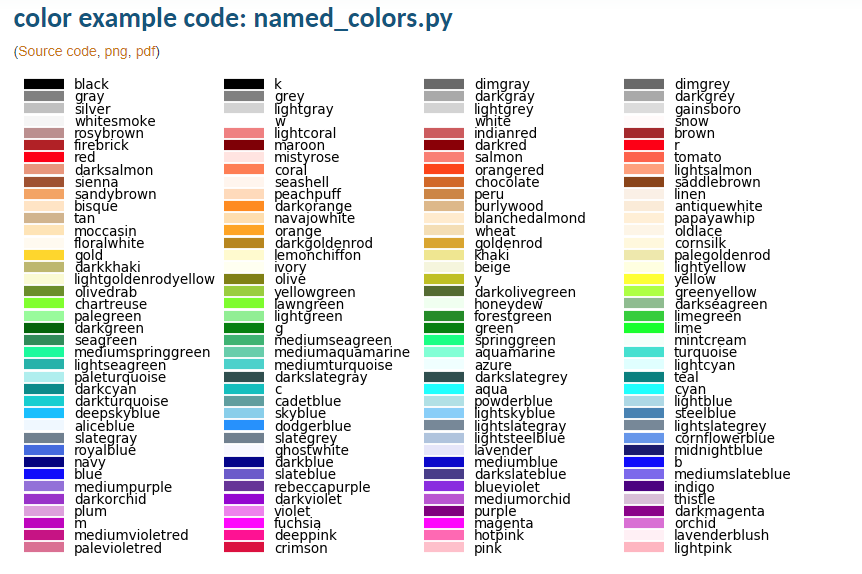## Python Matplotlib画图基础介绍

### 文章目录

Matplotlib 是 Python 中最常用的一个绘图库，主要用于绘制各种图形，包括散点图、柱状图、3D图、等高线图等等。在做研究过程中肯定会经常用，本文做一个简单的入门介绍，也给出极佳的参考手册，以备查用。内容主要参考了的内容，我自己用jupyter notebook实现了一遍，我会给出所有源码的文件。# Matplotlib第一个简单的图形

%matplotlib inline
import numpy as np
import matplotlib.pyplot as plt

plt.figure(0)
X = np.random.normal(size=(12, 2))
#plt.scatter(X[:, 0], X[:, 1])
plt.plot(X[:,0],X[:,1],'mo') #m表示紫色，o表示小圆点，更多颜色见文末
plt.show()# 坐标轴控制，标题

# create a new figure
plt.figure(1)
x = np.linspace(0, 2 * np.pi, 50)
y = np.sin(x)
plt.plot(x, y,label="sin(x)")
plt.plot(x, y * 2,label="2sin(x)")
plt.title("sin(x) & 2sin(x)") # 图像上方的标题
plt.xlim((0, np.pi*2)) # 横坐标的数值范围
plt.ylim((-3, 3)) # 纵坐标的数值范围
plt.xticks(np.linspace(0,np.pi*2,5)) # 认为指定在坐标轴上显示什么数字，我用linspace来画出5个值
#plt.xticks((0, np.pi * 0.5, np.pi, np.pi * 1.5, np.pi * 2))
plt.xlabel('XXX')  # 标记横轴坐标名称
plt.ylabel('YYY')  # 标记纵轴坐标名称
plt.legend(loc='upper right') # 标记标签，loc属性表示标签的位置，不写loc的话就是默认best，其他见下文
plt.show()legend loc参数选项：# 打上文字标注，公式

plt.plot(x, y)

x0 = np.pi
y0 = 0

# 画出标注点
plt.scatter(x0, y0, s=50)

plt.annotate('sin($\pi$)=%s' % y0, xy=(np.pi, 0), xycoords='data', xytext=(+30, -30),
textcoords='offset points', fontsize=16,

plt.text(0.5, -0.25, "$TEXTsin(\pi)=0$", fontdict={'size': 16, 'color': 'r'})

plt.show()‘sin(np.pi)=%s’ % y0 代表标注的内容，可以通过字符串 %s 将 y0 的值传入字符串；

xytext=(+30, -30) 和textcoords='offset points’表示对于标注位置的描述 和 xy 偏差值，即标注位置是 xy 位置向右移动 30，向下移动30；
arrowprops 是对图中箭头类型和箭头弧度的设置，需要用 dict 形式传入。

# 一个图中画多张子图

plt.figure(2)
ax1 = plt.subplot(2, 2, 1) # （行，列，活跃区）
plt.plot(x, np.sin(x), 'r+')

ax2 = plt.subplot(2, 2, 2) # 独立的y轴
plt.plot(x, 2 * np.sin(x), 'go')

ax3 = plt.subplot(2, 2, 3)
plt.plot(x, np.cos(x), '-b')

ax4 = plt.subplot(2, 2, 4, sharey=ax3) # 与 ax3 共享y轴
plt.plot(x, 2 * np.cos(x), 'y.')

plt.show()# 大小不一的多张子图

plt.figure(3)
ax1 = plt.subplot(2, 1, 1) # （行，列，活跃区）
plt.plot(x, np.sin(x), '-r+')

ax2 = plt.subplot(2, 3, 4)
plt.plot(x, 2 * np.sin(x), 'g')

ax3 = plt.subplot(2, 3, 5, sharey=ax2)
plt.plot(x, np.cos(x), 'b')

ax4 = plt.subplot(2, 3, 6, sharey=ax2)
plt.plot(x, 2 * np.cos(x), 'y')

plt.show()# 散点图

k = 500
x = np.random.rand(k)
y = np.random.rand(k)
size = np.random.rand(k) * 50 # 生成每个点的大小
colour = np.arctan2(y, x) # 生成每个点的颜色大小
plt.scatter(x, y, s=size, c=colour)
plt.colorbar() # 添加颜色栏

plt.show()# 柱状图

k = 10
x = np.arange(k)
y = np.random.rand(k)
plt.bar(x, y) # 画出 x 和 y 的柱状图

# 增加数值
for x, y in zip(x, y):
plt.text(x, y , '%.2f' % y, ha='center', va='bottom')

plt.show()# plt.rcParams参数设置

plt（matplotlib.pyplot）使用rc配置文件来自定义图形的各种默认属性，称之为rc配置或rc参数。# 详细颜色字母对照表# 参考资料

 https://www.jianshu.com/p/c41ac57cea33
 https://blog.csdn.net/weixin_39010770/article/details/88200298
 Matplotlib可视化及应用
 https://matplotlib.org/gallery.html